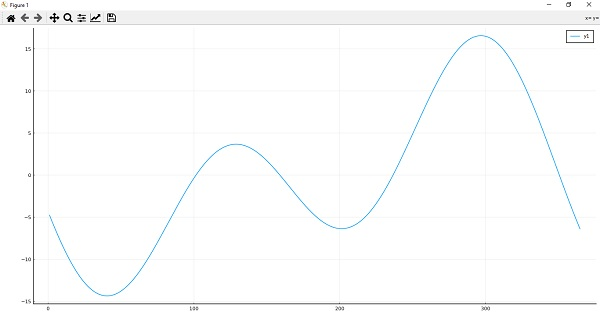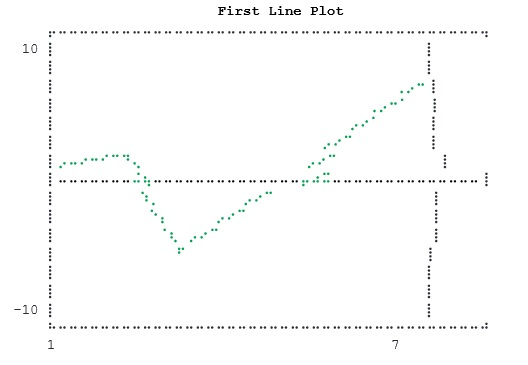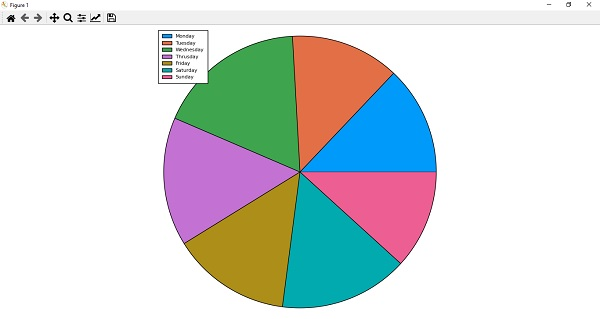# Julia Programming - Plotting

Julia has various packages for plotting and before starting making plots, we need to first download and install some of them as follows −

(@v1.5) pkg> add Plots PyPlot GR UnicodePlots


The package Plots is a high-level plotting package, also referred to as ‘back-ends’ interfaces with other plotting packages. To start using the Plots package, type the following command −

julia> using Plots
[ Info: Precompiling Plots [91a5bcdd-55d7-5caf-9e0b-520d859cae80]


## Plotting a function

For plotting a function, we need to switch back to PyPlot back-end as follows −

  julia> pyplot()
Plots.PyPlotBackend()


Here we will be plotting the equation of Time graph which can be modeled by the following function −

julia> eq(d) = -7.65 * sind(d) + 9.87 * sind(2d + 206);
julia> plot(eq, 1:365)
sys:1: MatplotlibDeprecationWarning: Passing the fontdict parameter of _set_ticklabels() positionally is deprecated since Matplotlib 3.3; the parameter will become keyword-only two minor releases later.
sys:1: UserWarning: FixedFormatter should only be used together with FixedLocator## Packages

Everyone wants a package that helps them to draw quick plots by text rather than graphics.

### UnicodePlots

Julia provides one such package called UnicodePlots which can produce the following −

• scatter plots

• line plots

• bar plots

• staircase plots

• histograms

• sparsity patterns

• density plots

We can add it to our Julia installation by the following command −

(@v1.5) pkg> add UnicodePlots


Once added, we can use this to plot a graph as follows:

julia> using UnicodePlots


### Example

Following Julia example generates a line chart using UnicodePlots.

julia> FirstLinePlot = lineplot([1, 2, 3, 7], [1, 2, -5, 7], title="First Line Plot", border=:dotted) First Line Plot### Example

Following Julia example generates a density chart using UnicodePlots.

Julia> using UnicodePlots
Julia> FirstDensityPlot = densityplot(collect(1:100), randn(100), border=:dotted)## VegaLite

This Julia package is a visualization grammar which allows us to create visualization in a web browser window. With this package, we can −

• describe data visualization in a JSON format

• generate interactive views using HTML5 Canvas or SVG

It can produce the following −

• Area plots

• Bar plots/Histograms

• Line plots

• Scatter plots

• Pie/Donut charts

• Waterfall charts

• Worldclouds

We can add it to our Julia installation by following command −

(@v1.5) pkg> add VegaLite


Once added we can use this to plot a graph as follows −

julia> using VegaLite


### Example

Following Julia example generates a Pie chart using VegaLite.

julia> X = ["Monday", "Tuesday", "Wednesday", "Thrusday", "Friday","Saturday","Sunday"];

julia> Y = [11, 11, 15, 13, 12, 13, 10]
7-element Array{Int64,1}:
11
11
15
13
12
13
10

julia> P = pie(X,Y)